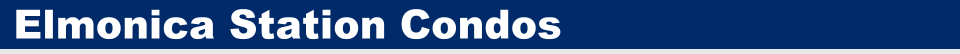Delightful Decimals and Perfect Percents Don't Just Learn Decimals and Percents ...Master Them!

 Delightful Decimals and Perfect Percents Don't Just Learn Decimals and Percents ...Master Them! Brimming with fun and educational games and activities, the Magical Math series provides everything you need to know to become a master of mathematics! In each of these books, Lynette Long uses her unique style to help you truly understand mathematical concepts as you play with everyday objects such as playing cards, dice, coins, and paper and pencil. In Delightful Decimals and Perfect Percents, you'll learn how to read and write decimals, how to change decimals into fractions and percents, and much more. While you play exciting games like the fast-paced Dynamite Decimal Reduction and Here's a Tip, you'll also learn to estimate percentages in your head and even figure out what tip to leave at a restaurant. And with great games like Zeros Exchange, Multiplication War, and Math Review, you'll practice adding, subtracting, multiplying, and dividing both decimals and percents - and have fun while you're doing it! So why wait? Jump right in and find out how easy it is to become a mathematics master!

 Percentage Calculator Learn and Calculate percentages online for free here! Percentage Calculator Video Learn about Percentage Calculator in this Video. Math 24 Game Questions and Solutions to all Math 24 Games. Fractions, Decimals, and Percents Math Workbook Develop proficiency in their conversions between fractions, decimals, and percentages. Smart Guide To Practical Math Smart Guide to Practical Math is a book designed to enable anyone who can use a calculator to answer a large number of questions that commonly arise in everyday life. Fraction Calculator This fraction calculator is recommended by teachers and is useful for all ages. Auto Loan Calculator Calculate auto loan payments. Maybe it is better to pay cash? Mortage Payment Calculator Time to refinance your home? Calculate your monthly payments based on interest rate and life of loan. Fractions, Percentages and Ratio Understand that 'percentage' means 'parts per 100', and that it can be used for conversions, comparisons and calculations. Fractions, Percentages, and Decimals Understanding fractions, decimals and percentages is necessary for success. Percentage Calculator Go here if you are looking for the best free online percentage calculator.

 Contact  |   Pacific City  |   Oregon Coast  |   World Information  |   Moon Information  |   Tic-Tac-Toe